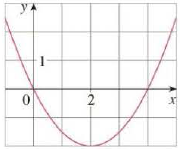Chapter 10.5, Problem 10E

Chapter
Section
Textbook Problem

Find an equation of the parabola. Then find the focus and directrix.10.To determine

To Find: The equation of the parabola, focus and the directrix by using figure (1).Explanation

Given:

By referring the figure (1), we can find the value of vertex.  Then the vertex is (2,2).

Calculation:

By referring the figure (1) graph is open up upward in the direction of y, so the equation,

x2=4py (1)

And the focus of the parabola is to be (0,p).

Compute the value of the p in equation below.

(xh)2=4p(yk) (2)

Substitute the value 2 for h and 2 for k in the equation (1).

(xh)2=4p(yk)(x2)2=4p(y(2))

(x2)2=4p(y+2) (3)

Substitute the value 0 for x and 0 for y in the equation (3).

(02)2=4p(0+2)4=8pp=12

Compute the focus of the parabola:

Focus=(h,k+p)

Substitute the value 12 for p2 for h and 2 for k

Still sussing out bartleby?

Check out a sample textbook solution.

See a sample solution

The Solution to Your Study Problems

Bartleby provides explanations to thousands of textbook problems written by our experts, many with advanced degrees!

Get Started

Expand each expression in Exercises 122. (y1y)2

Finite Mathematics and Applied Calculus (MindTap Course List)

In Problems 1-6, find the absolute maxima and minima for on the interval .

Mathematical Applications for the Management, Life, and Social Sciences

An integral for the solid obtained by rotating the region at the right about the x-axis is:

Study Guide for Stewart's Single Variable Calculus: Early Transcendentals, 8th

Find for y defined implicity by .

Study Guide for Stewart's Multivariable Calculus, 8th

Graph each ellipse. x216+(y+2)236=1

College Algebra (MindTap Course List)